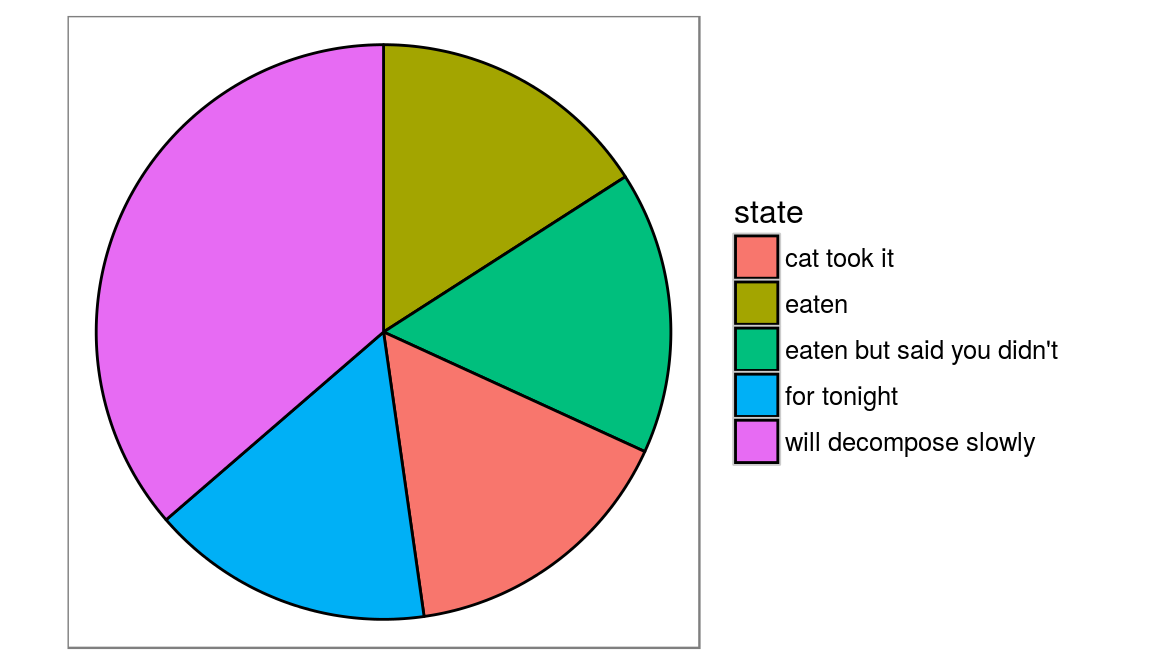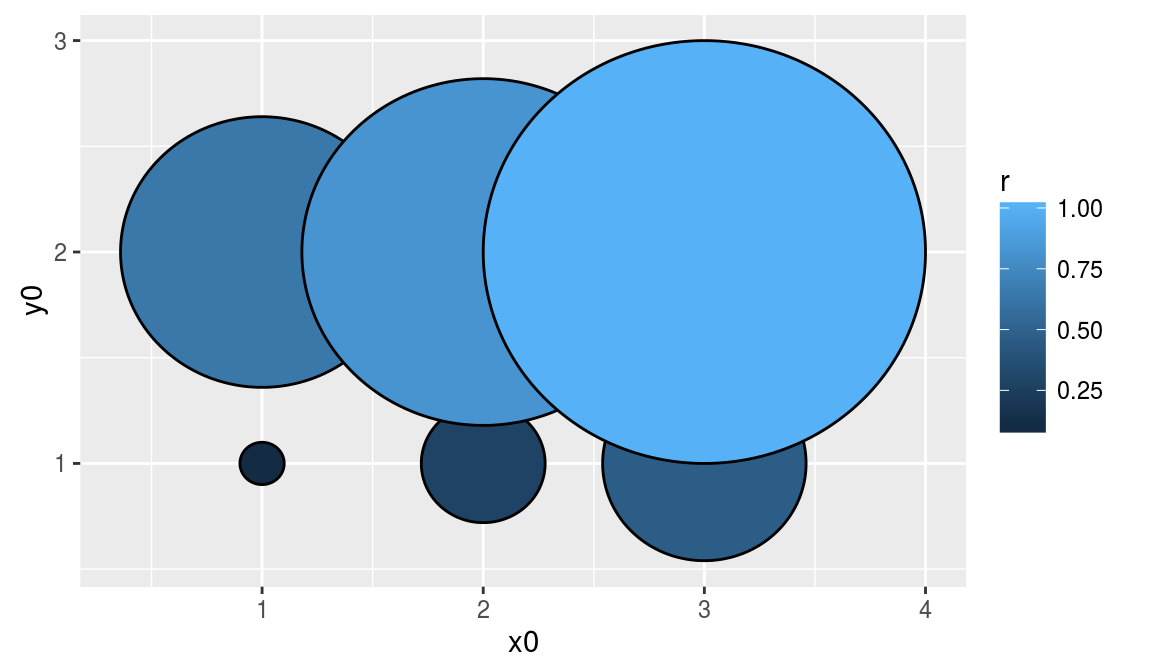ggplot2 now has an official extension mechanism. This means that others can now easily create their own stats, geoms and positions, and provide them in other packages. This should allow the ggplot2 community to flourish, even as less development work happens in ggplot2 itself. This page showcases these extensions.

### ggforce

https://github.com/thomasp85/ggforce

ggforce is aimed at providing missing functionality to ggplot2 through the extension system introduced with ggplot2 v2.0.0.

``````# Example from https://github.com/thomasp85/ggforce
library(ggplot2)
library(ggforce)

# We'll start by defining some dummy data
pie <- data.frame(
state = c('eaten', 'eaten but said you didn\'t', 'cat took it',
'for tonight', 'will decompose slowly'),
focus = c(0.2, 0, 0, 0, 0),
start = c(0, 1, 2, 3, 4),
end = c(1, 2, 3, 4, 2*pi),
amount = c(4,3, 1, 1.5, 6),
stringsAsFactors = FALSE
)

p <- ggplot() + theme_no_axes() + coord_fixed()

# For low level control you define the start and end angles yourself
p + geom_arc_bar(aes(x0 = 0, y0 = 0, r0 = 0, r = 1, start = start, end = end,
fill = state),
data = pie)````````````# Here are some data describing some circles
circles <- data.frame(
x0 = rep(1:3, 2),
y0 =  rep(1:2, each=3),
r = seq(0.1, 1, length.out = 6)
)
ggplot() + geom_circle(aes(x0=x0, y0=y0, r=r, fill=r), data=circles)``````